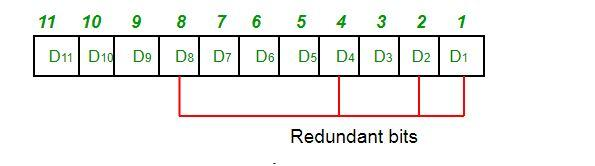Home Technology Redundant bit

# Redundant bit

The same algorithm for the ring redundancy code is checked.

If there is an error, it needs to be retransmitted.

2. Examples of cyclic redundancy code generation and code word correctness verification.

Analysis of the CRC check code algorithm The coding method of the CRC check code is to divide the binary data t(x) to be sent by the generating polynomial g(x), and use the final remainder as the CRC check code .

The implementation steps are as follows:

(1) Suppose the data block to be sent is an m-bit binary polynomial t(x), and (2) the generator polynomial is an r-order g( x). Add

to the end of the data block by adding r zeros, (3) the length of the data block is increased to m+r bits, and (4) the corresponding binary polynomial is.

(5) Divide with the generator polynomial g(x), (6) Find the remainder as the binary polynomial y(x) of order r-1. This binary polynomial y(x

) is the CRC check code of t(x) encoded by the generator polynomial g(x).

(7) Subtract y(x) by modulo 2, and (8) get a binary polynomial. It is the string to be sent that contains the CRC check code.

As can be seen from the CRC encoding rules, CRC encoding actually converts the m-bit binary polynomial t(x) sent on behalf of the generation to be divisible by g(x)

Is a binary polynomial with m+r bits, so the received data can be used to remove g(x) when decoding. If the remainder is zero, it means there is no error in the transmission process.If the remainder is not zero, Then there must be an error in the transmission process. Many CRC hardware decoding circuits perform error detection in this way.

At the same time, it can be regarded as a combination of t(x) and CRC check code, so when decoding the received binary data, remove the tail r-bit data to obtain

It is the original data.

In order to understand the CRC check code encoding process more clearly, a simple example is used below to illustrate the CRC check code encoding process. Because the coding process of CRC-32,

CRC-16, CCITT and CRC-4 is basically the same, only the number of bits is different from the generating polynomial.

Redundant bit-the working principle of cyclic redundancy code Cyclic redundancy code CRC can be obtained by using the pre-appointed generator polynomial G(X) when encoding at the transmitting end and checking at the receiving end, K The information bit to be sent

can correspond to a (k-1)

polynomial of degree K(X), and the r-bit redundant bit corresponds to a (r-1) Polynomial of degree R(X), n=k+r-bit code word composed of r-bit redundant bits corresponds to one

(n-1)-degree polynomial T(X)=Xr*K (X)+R(X).

.Characteristics of cyclic redundancy check code

1) All odd bit errors can be detected;

2) All double bit errors can be detected ;

3) All burst errors less than or equal to the length of the check bit can be detected.